# ML Aggarwal Solutions for Class 6 Maths Chapter 6 Fractions

ML Aggarwal Solutions for Class 6 Maths Chapter 6 Fractions are provided here. Practising ML Aggarwal Solutions on a daily basis helps students to solve the complex problems effortlessly and get their doubts cleared. Students of Class 6 are suggested to solve ML Aggarwal Solutions for Class 6 to strengthen their fundamentals and be able to solve questions that are usually asked in the examination.

Chapter 6 – Fractions is a number representing a part of a whole. This whole may be a single object or a group of objects. In the ML Aggarwal Solutions for Class 6 Maths Chapter 6, students are going to learn some topics, which are, various types of fractions, method of changing unlike fractions to like fractions, addition and subtraction of fractions, multiplication of fractions, reciprocal of fractions.

## Download the PDF of ML Aggarwal Solutions for Class 6 Maths Chapter 6 Fractions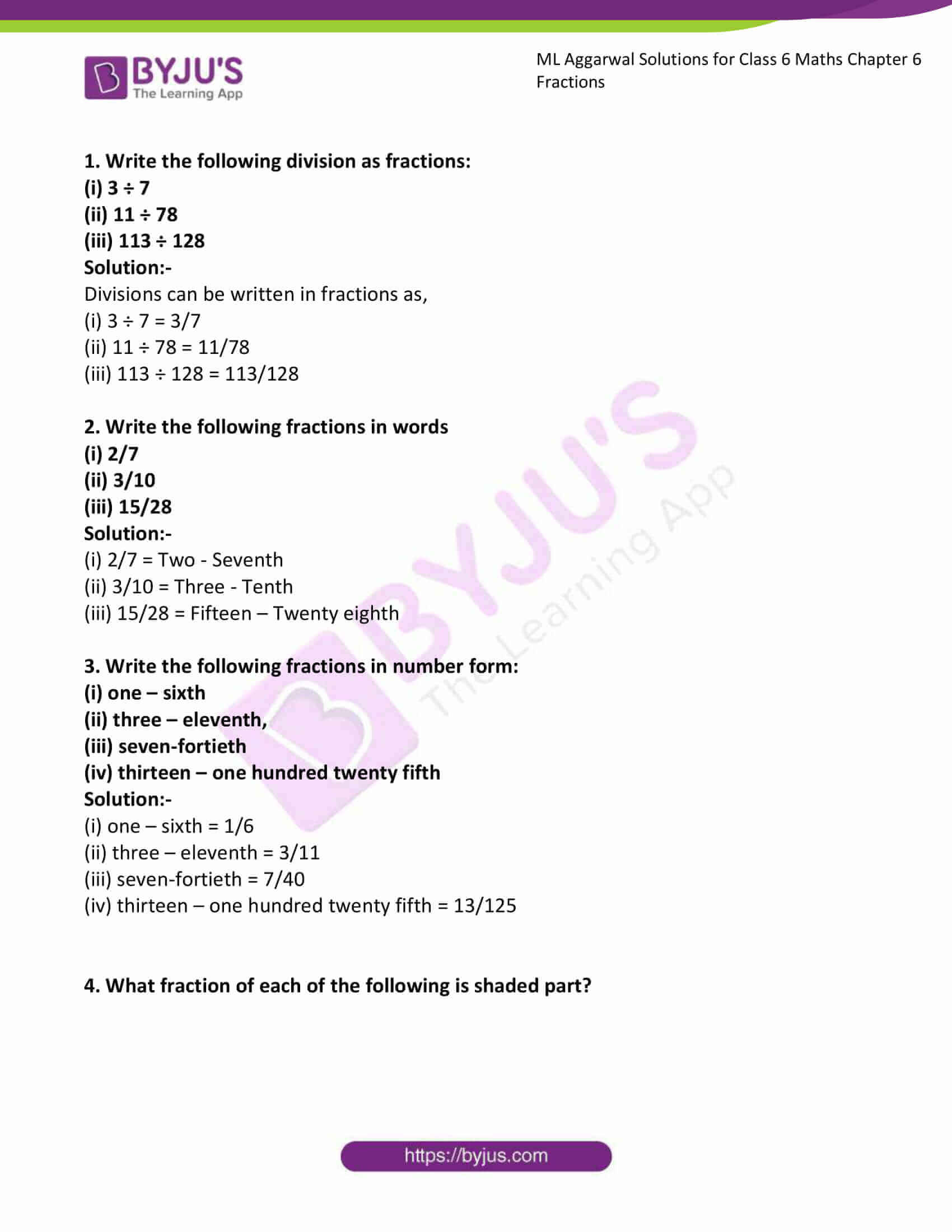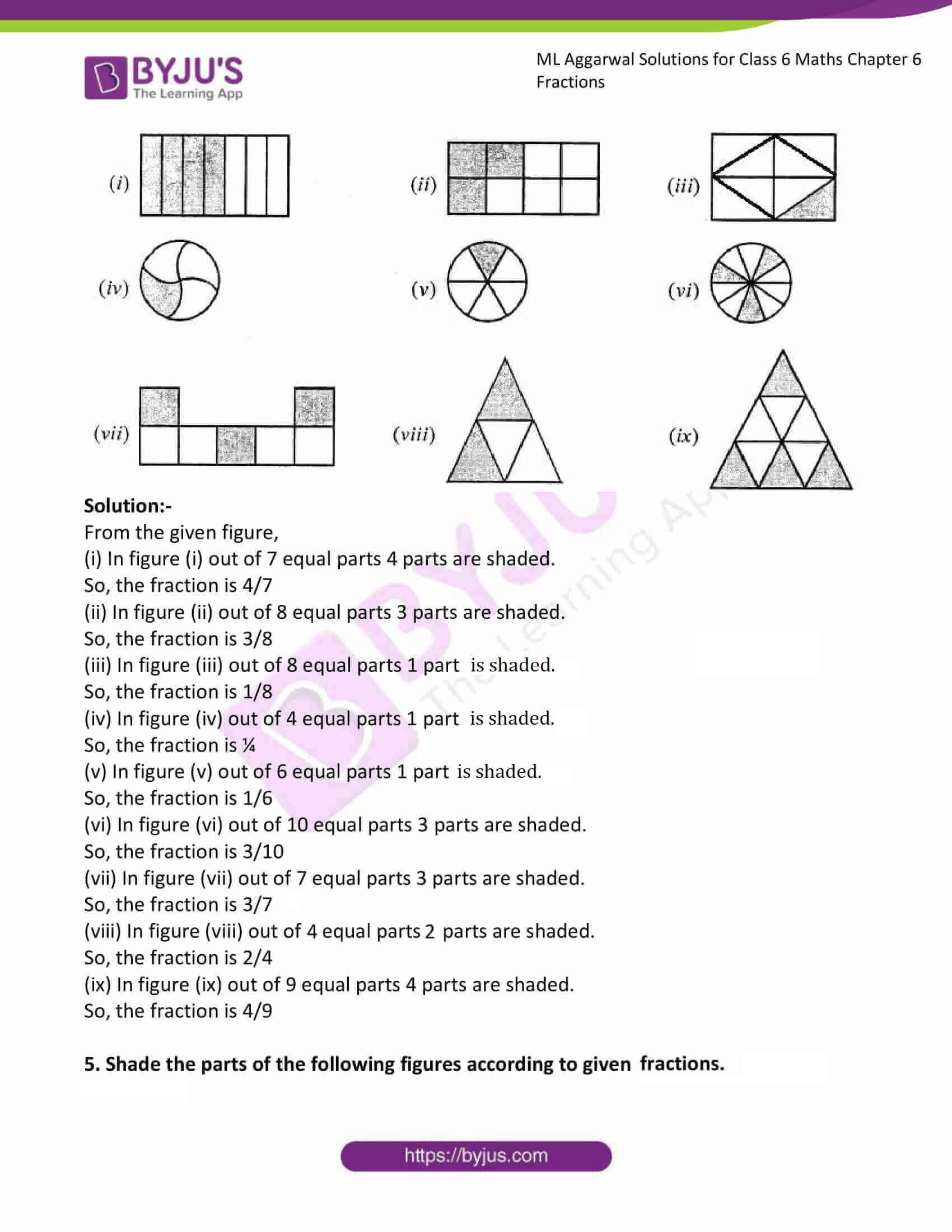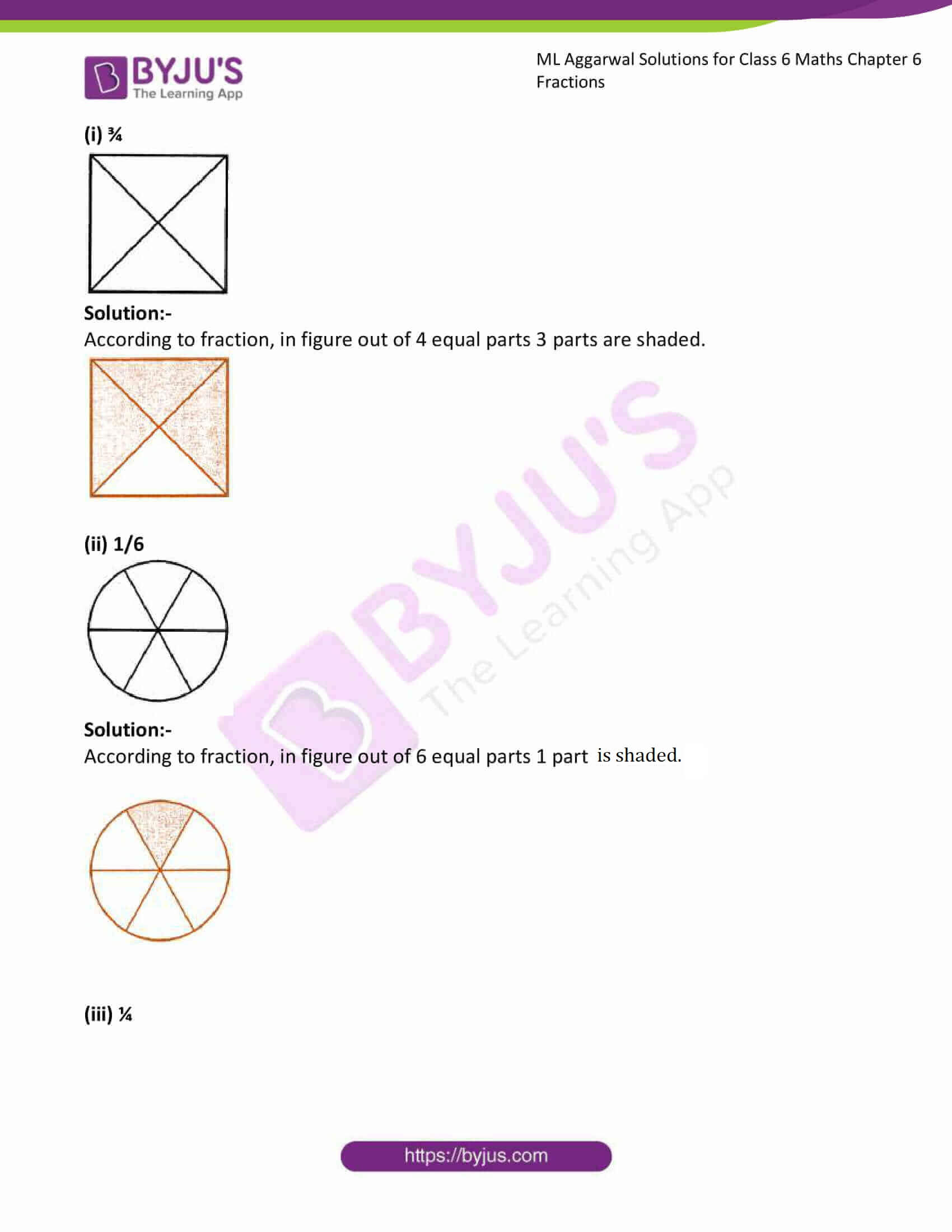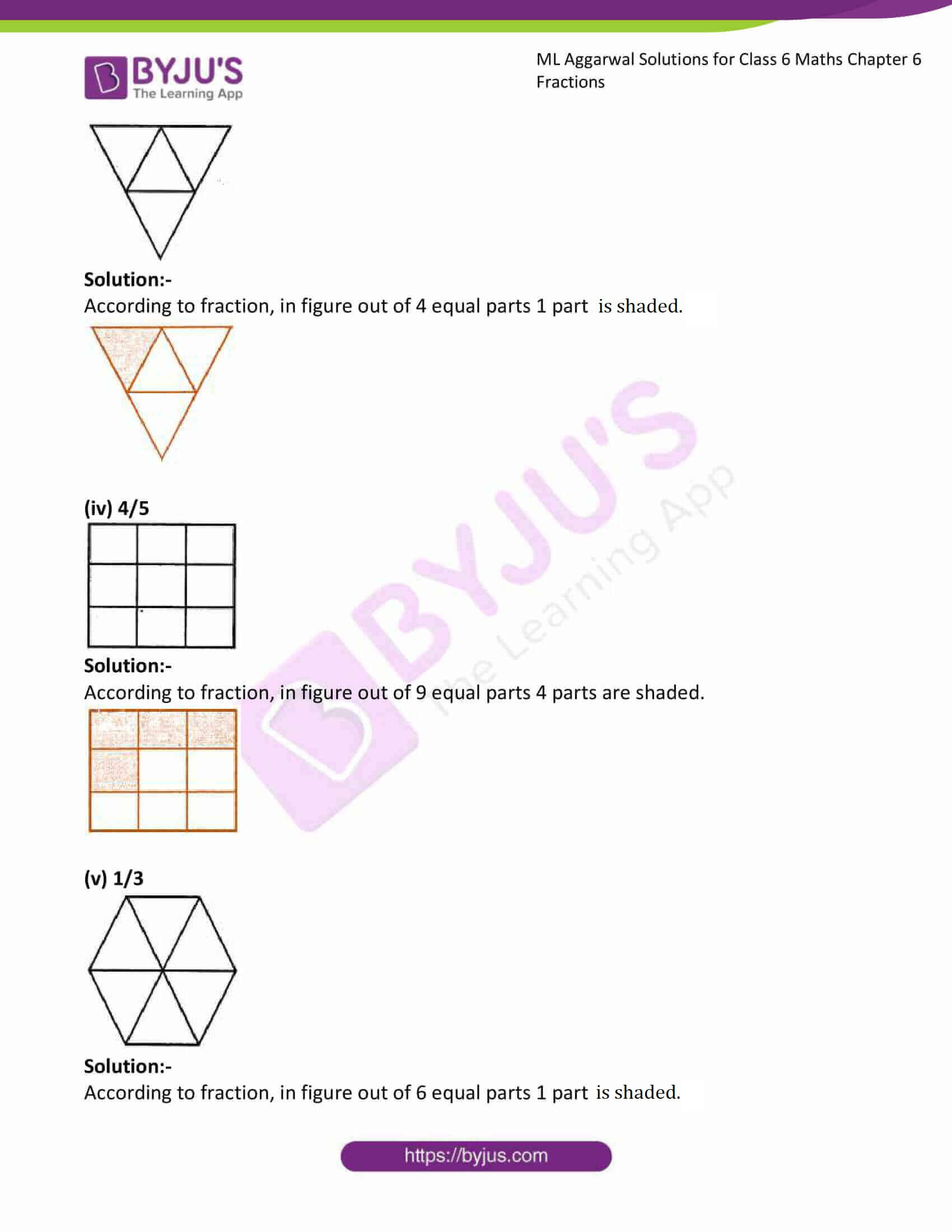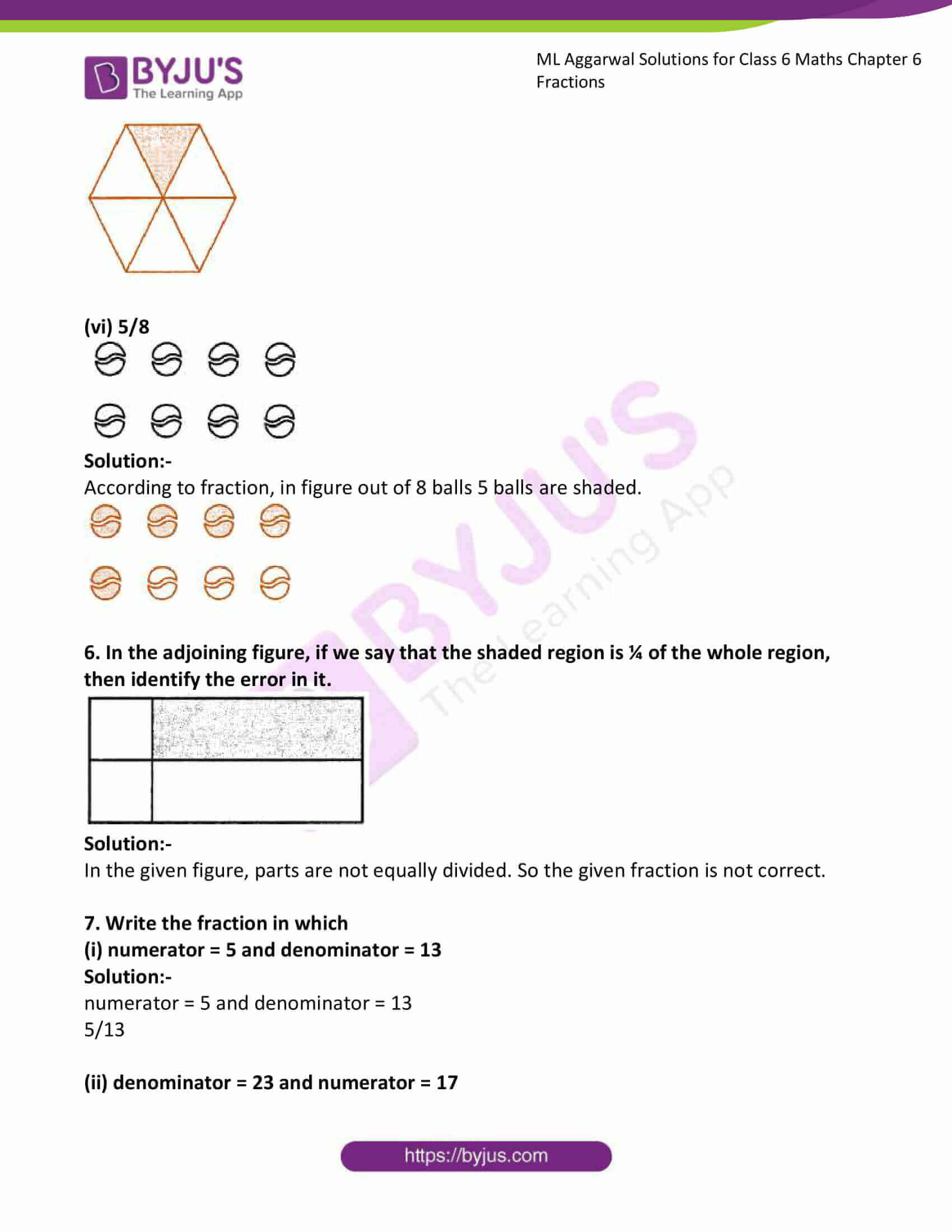### Access answers to ML Aggarwal Solutions for Class 6 Maths Chapter 6 Fractions

1. Write the following division as fractions:
(i) 3 ÷ 7
(ii) 11 ÷ 78
(iii) 113 ÷ 128

Solution:-

Divisions can be written in fractions as,

(i) 3 ÷ 7 = 3/7

(ii) 11 ÷ 78 = 11/78

(iii) 113 ÷ 128 = 113/128

2. Write the following fractions in words

(i) 2/7

(ii) 3/10

(iii) 15/28

Solution:-

(i) 2/7 = Two – Seventh

(ii) 3/10 = Three – Tenth

(iii) 15/28 = Fifteen – Twenty eighth

3. Write the following fractions in number form:
(i) one – sixth
(ii) three – eleventh,
(iii) seven-fortieth
(iv) thirteen – one hundred twenty fifth

Solution:-

(i) one – sixth = 1/6

(ii) three – eleventh = 3/11

(iii) seven-fortieth = 7/40

(iv) thirteen – one hundred twenty fifth = 13/125

4. What fraction of each of the following is shaded part?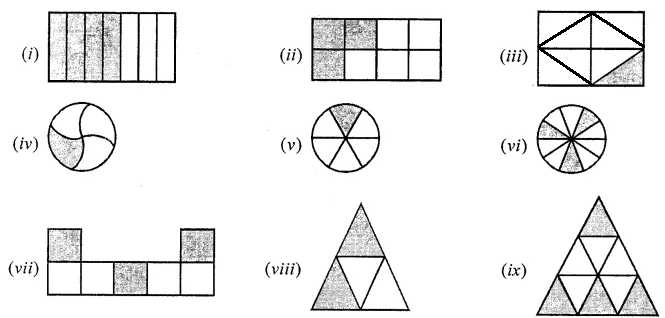Solution:-

From the given figure,

(i) In figure (i) out of 7 equal parts 4 parts are shaded.

So, the fraction is 4/7

(ii) In figure (ii) out of 8 equal parts 3 parts are shaded.

So, the fraction is 3/8

(iii) In figure (iii) out of 8 equal parts 1 part is shaded.

So, the fraction is 1/8

(iv) In figure (iv) out of 4 equal parts 1 part is shaded.

So, the fraction is ¼

(v) In figure (v) out of 6 equal parts 1 part is shaded.

So, the fraction is 1/6

(vi) In figure (vi) out of 10 equal parts 3 parts are shaded.

So, the fraction is 3/10

(vii) In figure (vii) out of 7 equal parts 3 parts are shaded.

So, the fraction is 3/7

(viii) In figure (viii) out of 4 equal parts 2 parts are shaded.

So, the fraction is 2/4

(ix) In figure (ix) out of 9 equal parts 4 parts are shaded.

So, the fraction is 4/9

5. Shade the parts of the following figures according to given fractions.

(i) ¾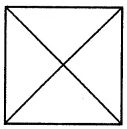Solution:-

According to fraction, in figure out of 4 equal parts 3 parts are shaded.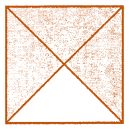(ii) 1/6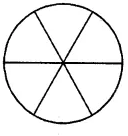Solution:-

According to fraction, in figure out of 6 equal parts 1 part is shaded.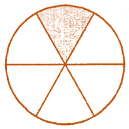(iii) ¼Solution:-

According to fraction, in figure out of 4 equal parts 1 part is shaded.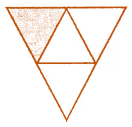(iv) 4/5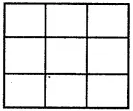Solution:-

According to fraction, in figure out of 9 equal parts 4 parts are shaded.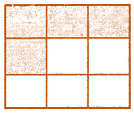(v) 1/3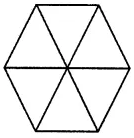Solution:-

According to fraction, in figure out of 6 equal parts 1 part is shaded.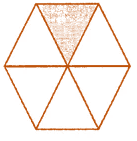(vi) 5/8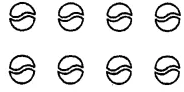Solution:-

According to fraction, in figure out of 8 balls 5 balls are shaded.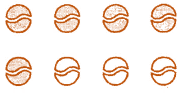6. In the adjoining figure, if we say that the shaded region is ¼ of the whole region, then identify the error in it.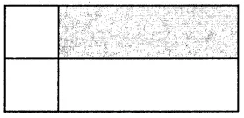Solution:-

In the given figure, parts are not equally divided. So the given fraction is not correct.

7. Write the fraction in which
(i) numerator = 5 and denominator = 13

Solution:-

numerator = 5 and denominator = 13

5/13

(ii) denominator = 23 and numerator = 17

Solution:-

denominator = 23 and numerator = 17

17/23